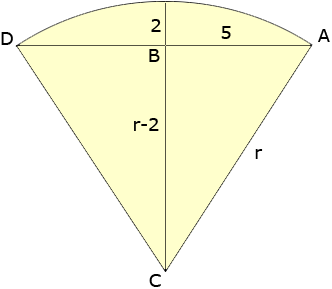Question: I know the base length of my arc (10 inches) - I also know the height at the center to the arc (2 inches). I don't think the end is at the midpoint tho. How do I figure out how long the arc length is? My question involves being able to cut a round pipe into an arc that is 10 inches wide and 2 inches tall. I need to know the smallest diameter pipe to buy in order to fulfill these requirements. Please help!?! Thank you in advance. Hi, I am not sure I understand your question but I assumed that you have a circular arc. Let us know if we haven't interpreted the question correctly. I drew a diagram, labeled the radius r an drew a line from the centre C to the midpoint of the arc.The triangle ABC is a right triangle so, by Pythagoras theorem, 52 + (r - 2)2 = r2, or 25 + r2 - 4r + 4 = r2, or r = 29/4 = 7.25 inches. Thus you need pipe with a diameter of 14.5 inches. If you need to find the length of the arc then that involves some trigonometry. cos(BCA) = (r - 2)/r = 5.25/7.25 = 0.7241 and hence the measure of angle BCA is cos-1(0.7241) = 0.7610 radians and thus the measure of angle DCA is 20.7610 = 1.522 radians. The length a of the arc is given by a = rangle(DCA) = 7.251.522 = 11.42 inches o almost 11.5 inches. Penny